## 高精半导体芯片激光切割控制系统

1、系统选型

（1）惯量计算

X 轴负载惯量：J x =Mx(P /2π) 2

= 20.2×（0.005/2×3.14）

= 0.128×10 -4 kgm 2

Y 轴负载惯量：J y =My(P/2π) 2

=2.9×（0.005/2×3.14） 2

= 0.0184×10 -4 kgm 2

= 0.405×10 -4 kgm 2

X 轴电机负载惯量： J LX = J x + J 2 =0.533×10 -4 kgm 2

Y 轴电机负载惯量： J LY = J y + J 2 =0.4234×10 -4 kgm 2

400w 电机惯量：J m4 = 0.26×10 -4 kgm 2

750w 电机惯量：J m7= 0.87×10 -4 kgm 2

（2）扭矩计算

= 3000 × 2π / 60=314 rad

X 轴负载转矩：T LX =μMgP / 2πη

=0.2×20.2×9.8×0.005/2×3.14×0.9=0.035 Nm

Y 轴负载转矩：T LY =μMgP / 2πη

=0.2×2.9×9.8×0.005/2×3.14×0.9=0.00304 Nm

X 轴启动转矩：T px = (J LX + J m7 ) ● a + + T LX

= 0.441 + 0.035 = 0.476 Nm

Y 轴启动转矩：T py = (J LY + J m4 ) ● a + + T LY

= 0.215 + 0.00304 = 0.218 Nm

X 轴制动转矩：Tsx = (J LX + J m7 ) ● a + - T L

=0.441-0.035=0.406Nm

Y 轴制动转矩：Tsy = (J LY + J m4 ) ● a + - T L

= 0.215 - 0.00304 = 0.212 Nm

（3）盘式电机选型

1.1 实现功能

1、原点复归

2、激光功率检测

3、芯片切割

1.2 系统设计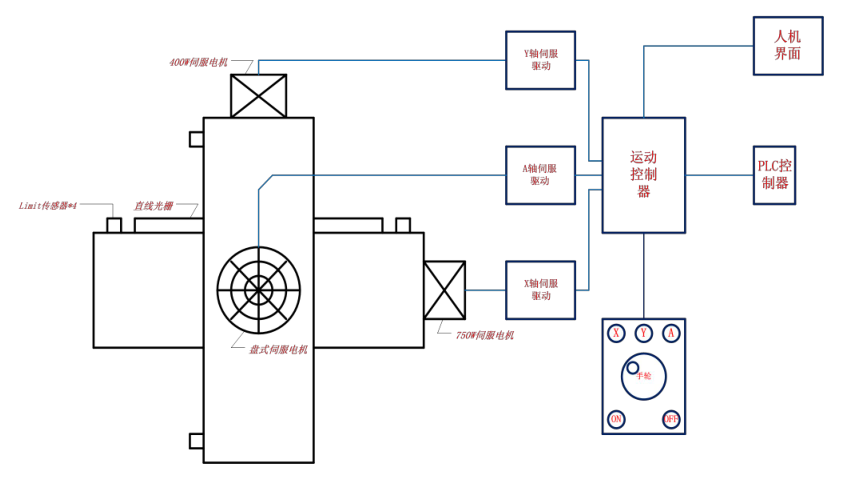1.3 设备图片

1.3 设备图片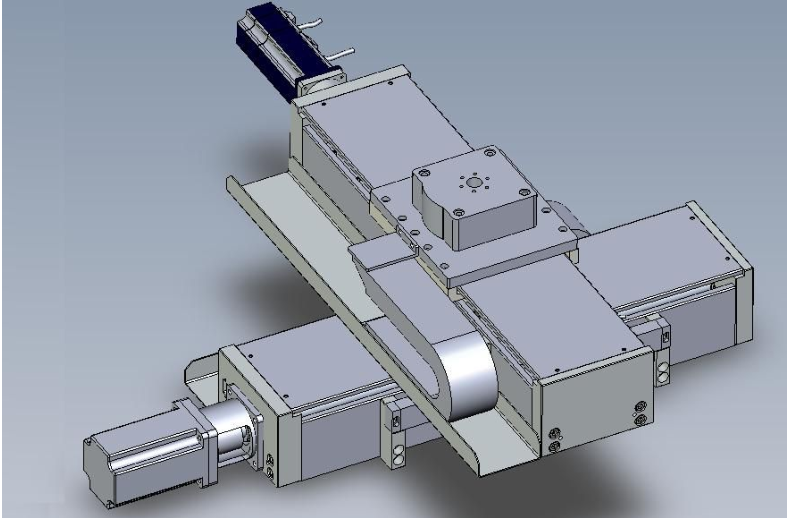2 控制过程

2 控制过程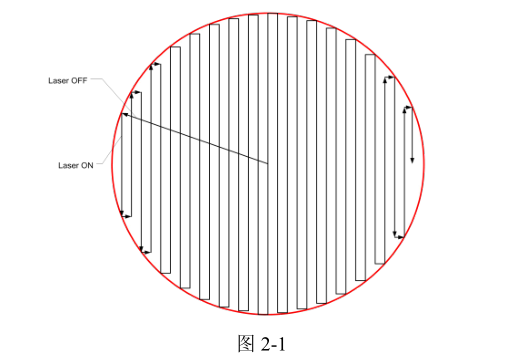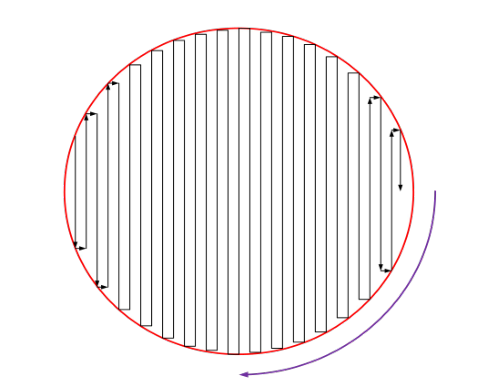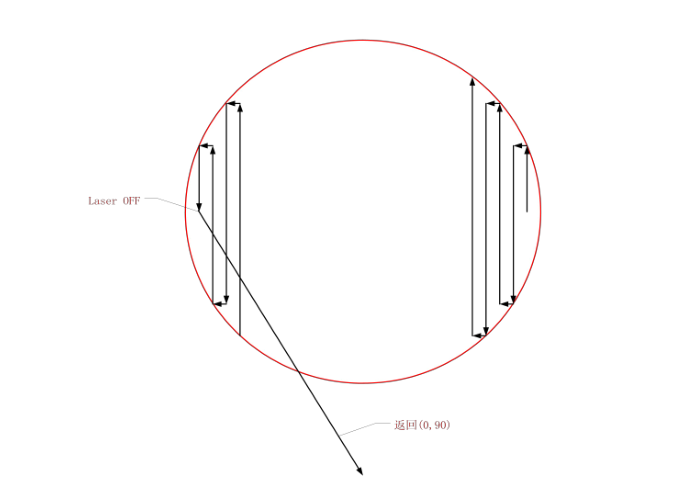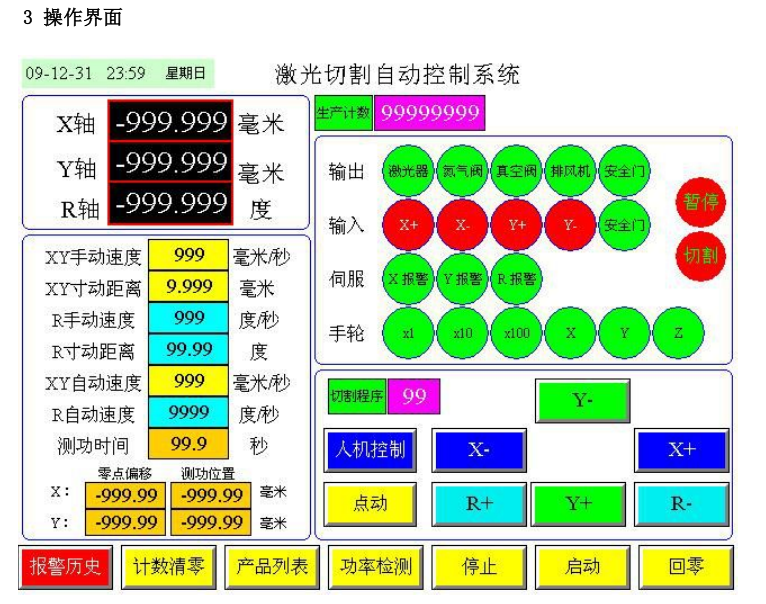4 结束语

May 10, 2019
May 10, 2019
May 10, 2019
May 10, 2019
May 10, 2019
May 10, 2019
May 10, 2019
May 10, 2019
May 10, 2019
May 10, 2019
May 10, 2019
May 10, 2019
May 10, 2019
May 10, 2019
May 10, 2019
##### 服务电话:
010-56298855-881#